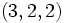# Dicyclic group:Dic12

Jump to: navigation, search
This article is about a particular group, i.e., a group unique upto isomorphism. View specific information (such as linear representation theory, subgroup structure) about this group
View a complete list of particular groups (this is a very huge list!)[SHOW MORE]

This group, sometimes denoted$\operatorname{Dic}_{12}$ and sometimes denoted$\Gamma(3,2,2)$, is defined in the following equivalent ways:

• It is the dicyclic group (i.e., the binary dihedral group) of order$12$, and hence of degree$3$.
• It is the binary von Dyck group with parameters$(3,2,2)$.

A presentation for the group is given by:$\langle a,b,c \mid a^3 = b^2 = c^2 = abc \rangle$.

## GAP implementation

### Group ID

This finite group has order 12 and has ID 1 among the groups of order 12 in GAP's SmallGroup library. For context, there are 5 groups of order 12. It can thus be defined using GAP's SmallGroup function as:

SmallGroup(12,1)

For instance, we can use the following assignment in GAP to create the group and name it$G$:

gap> G := SmallGroup(12,1);

Conversely, to check whether a given group$G$ is in fact the group we want, we can use GAP's IdGroup function:

IdGroup(G) = [12,1]

or just do:

IdGroup(G)

to have GAP output the group ID, that we can then compare to what we want.

### Other definitions

The group can also be defined using its presentation:

F := FreeGroup(3);
G := F/[F.1^3 * F.2^(-2), F.2^2 * F.3^(-2), F.1 * F.2 * (F.3)^(-1)];